# Selina Concise Mathematics Class 9 ICSE Solutions Construction of Polygons

## Selina Concise Mathematics Class 9 ICSE Solutions Construction of Polygons (Using ruler and compass only)

APlusTopper.com provides step by step solutions for Selina Concise Mathematics Class 9 ICSE Solutions Chapter 15 Construction of Polygons (Using ruler and compass only). You can download the Selina Concise Mathematics ICSE Solutions for Class 9 with Free PDF download option. Selina Publishers Concise Mathematics for Class 9 ICSE Solutions all questions are solved and explained by expert mathematic teachers as per ICSE board guidelines.

Selina ICSE Solutions for Class 9 Maths Chapter 15 Construction of Polygons (Using ruler and compass only)

Solution 1: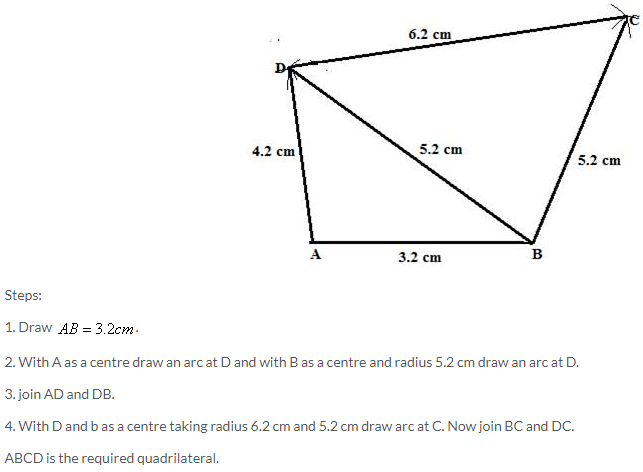Solution 2:Solution 3: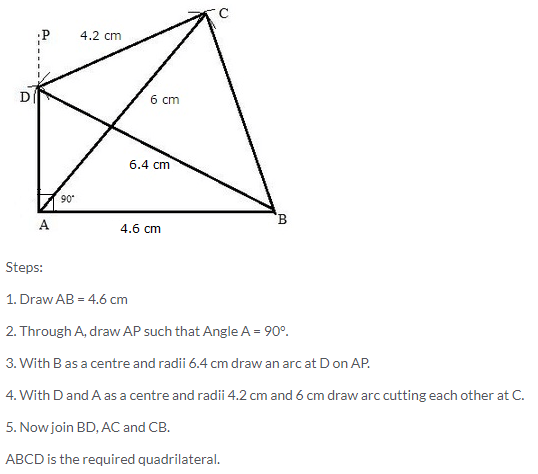Solution 4: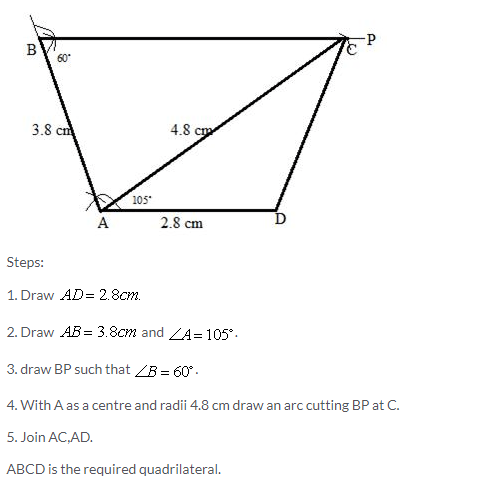Solution 5:Solution 6:Solution 7: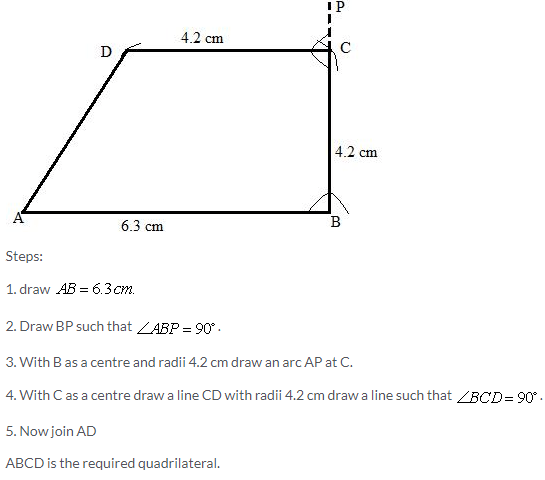Solution 8: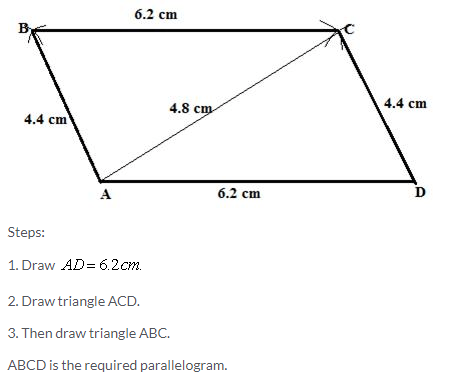Solution 9:Solution 10:Solution 11: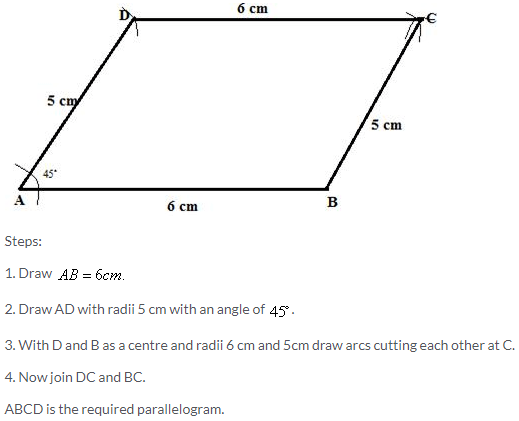Solution 12:Solution 13:Solution 14:Solution 15: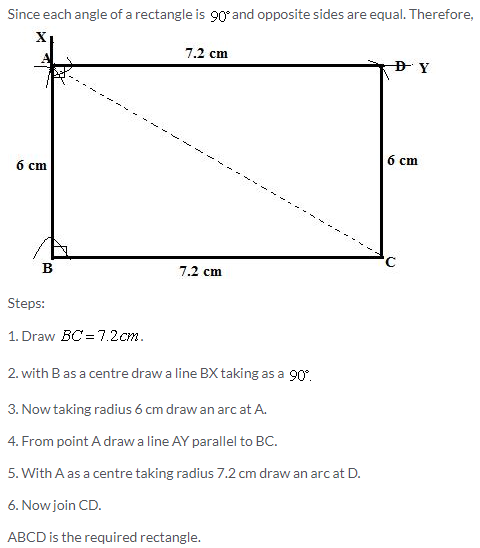Solution 16: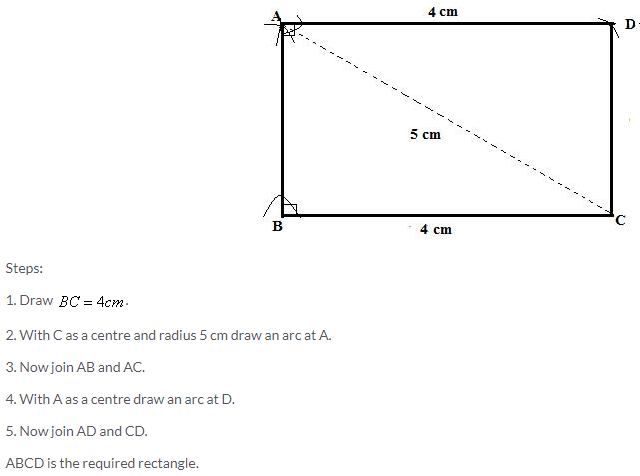Solution 17:Solution 18: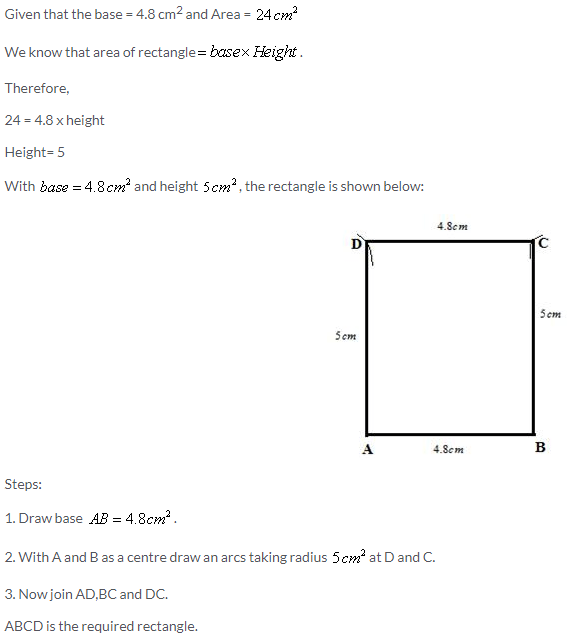Solution 19: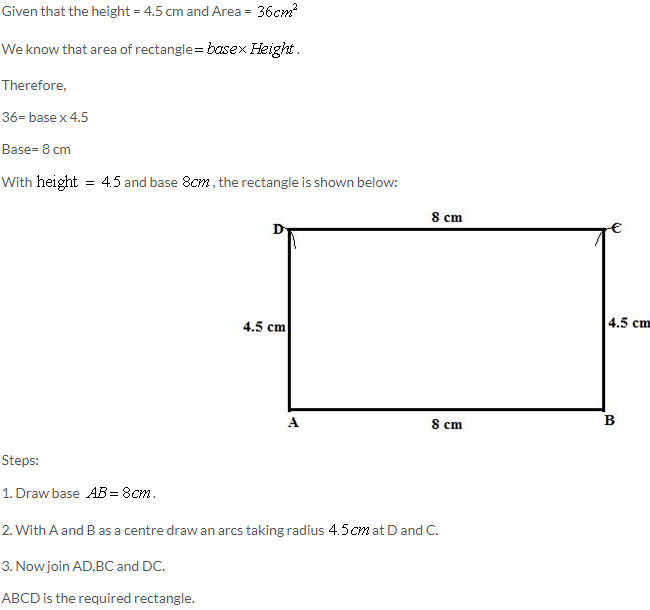Solution 20:Solution 21:Solution 22: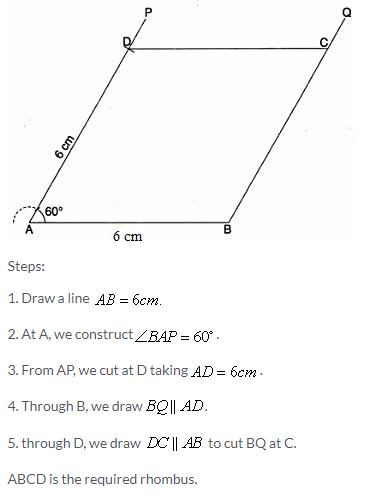Solution 23: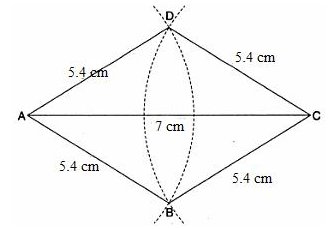Steps:

1. We construct the segment AC = 7cm.
2. With A as a centre and radius 5.4 cm , we draw an arc extending on both sides of AC.
3. With C as centre and same radius as in step 2, we draw an arc extending on both sides of AC to cut the first arc at B and D.
4. Join AB,BC,CD and DA.

ABCD is the required rhombus.

Solution 24:Solution 25:Solution 26: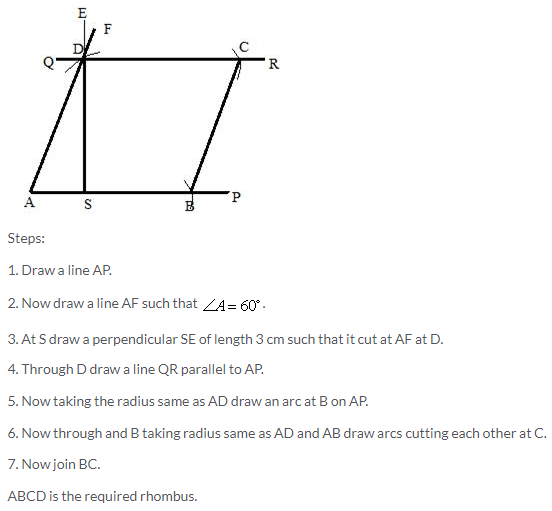Solution 27:Solution 28: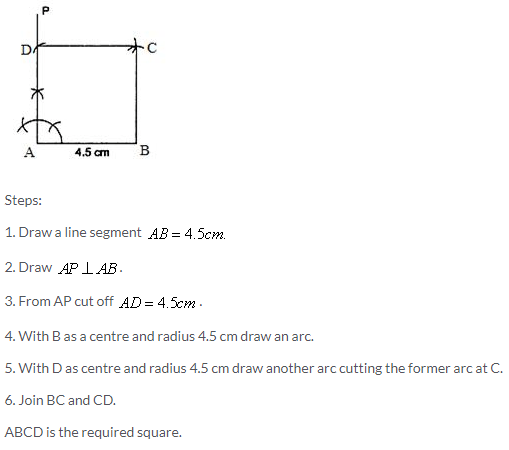Solution 29:Solution 30: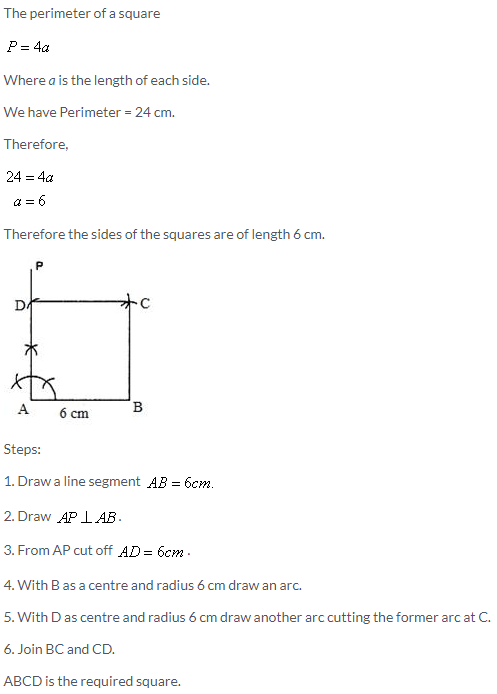Solution 31: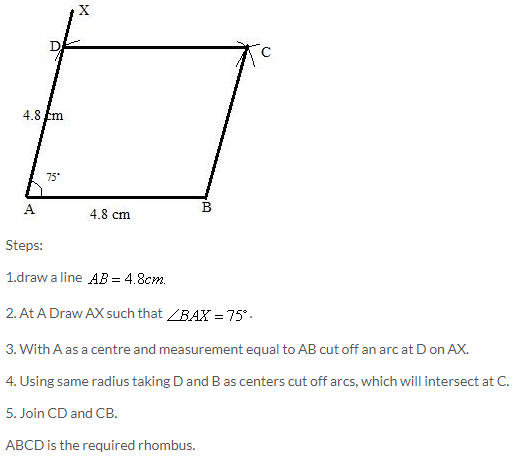Solution 32: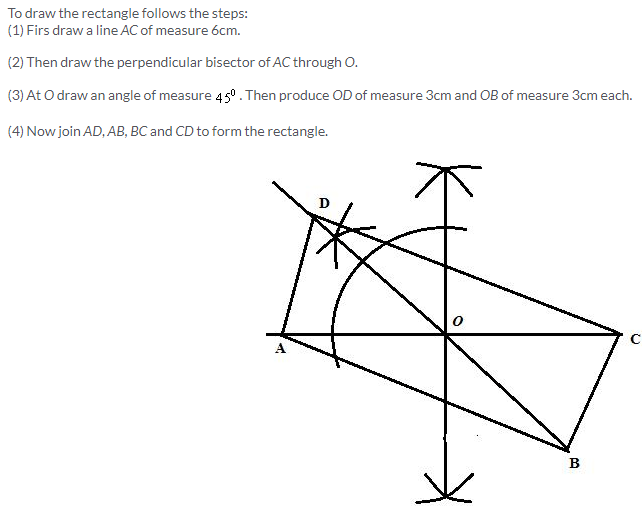Solution 33:Solution 34:Solution 35:Solution 36:Solution 37: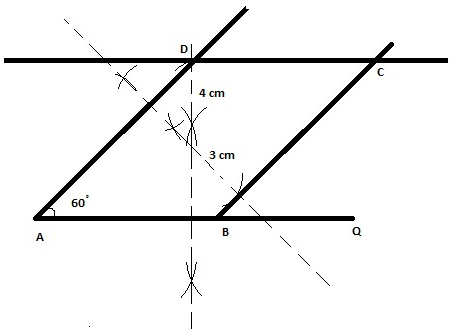Steps:

1. Draw a base line AQ.
2. From A take some random distance in compass and draw one are below and above the line. Now without changing the distance in compass draw one are below and above the line. These arcs intersect each other above and below the line.
3. Draw the line passing through these intersecting points, you will get a perpendicular to the line AQ.
4. Take distance of 4 cm in compass and mark an arc on the perpendicular above the line. Draw a line parallel to line AQ passing through through this arc.
5. From point A measure an angle of 60 degree and draw the line which intersect above drawn line at some point label it as D.
6. Using the procedure given in step 2 again draw a perpendicular to line AD.
7. Take distance of 3 cm in compass and mark an arc on the perpendicular above the line. Draw a line parallel to line AD passing through through this arc which intersect the line AQ at some point label it as B and to other line at point C.

ABCD is the required parallelogram.

Solution 38:
To draw the parallelogram follows the steps:

1. First draw a line AB of measure 6cm. Then draw an angle of measure 450 at point A such that ∠DAB = 450 and AD = 5cm.
2. Now draw a line CD parallel to the line AB of measure 6cm. Then join BC to construct the parallelogram as shown below:Solution 39:More Resources for Selina Concise Class 9 ICSE Solutions